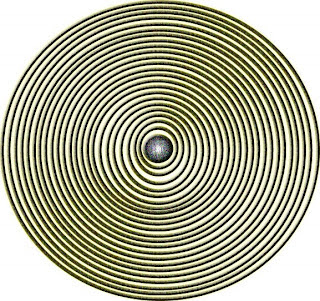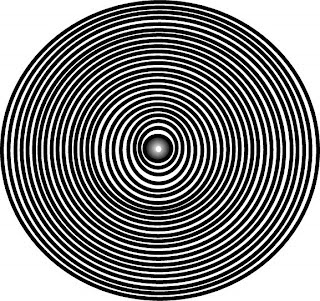## Wednesday, December 3, 2008

((((((((((((((((((()))))))))))))))))))))))))))))))))))((((((((((((((((((((((((((((((((((((((((((
౯౦()(()౦()౯)(౦౯()(౦(౦౯)౯)(౦౯౦)౯౦(౦౯)౯౦౯)౯౦(౯)(౦(౦౯౯౦౯౦౦(౦౯()౯౦౦౯)()(())౯౦౯౦౯౦
)(()()()()()()()()௯)௯)(0௯0௯0௯)௯)(0(0()௯)(0(0௯)௯)௯0(0௯)௯0()௯)(0()(
)()௯)௯0(0௯)௯0()௯)(௦௯௦௦ப௯௯௦௯௦())((0())(()0௯)()(())௯)()(0௯)(0(௦௯௦)(())
)()()()(௯0(00)௯)()(((௮()()(௯௮^%)௫\$0*()()()*௯)௯)௬)\$௩)#)((0௯௯0()(0()(
(\$##)(%^௯())(()௯)()\$௪)^^()()%(%((\$#௩0௯#)*()*)^^)*)*)**)*)%0*^*)0)()^௫௪\$
(())()()((((((((((((((((((((()))))))))((((()))))))))))))))))((((((((((((())))))))))((((((((((((()))))))))
((((((((()))))))))))))(((((((((())))))))))))(((((((((()))))))))((((((((()))))(((((()(((((()()((((((((((()
()(((((((௯)()()))))))))))))))))(0(0)())))))))))))))))))))())()௯))))))))))))))()(((((((((((((0௯()
((((((((((((()0௯(0)))))))))))))))))))))))))))))))))))0௯)0())))))))))))))))))0௯)())))))))))))))so
Five Card Nancy is a game that Scott McCloud invented வ்தேரே You get to resequence ப்ளைன் கோட் old Nancy panels according to your ( )'s ( ). Here's what I came ഉപ്(*%)( up with: : :आईटी'स: The Work of fArt in the Age Of Digital Reproduction : :It's getting damn near impossible to make dangerous or even relevent art in this desensitized culture of masshmedia jello for all.>>) The mold is both expanding and contracting and seems to've crystallized into some iphoney kind of thingi.:(Art, much like gnosis, is a dead term. Pure abstraction is absolutely impossible to realize in any context: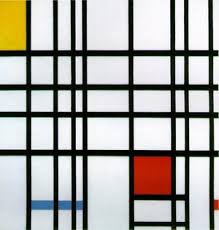Xpression has always been a thing of the past.News is the oldest oxymoron in the book.Good old fashioned Modernism has been sucked virtually dry;)))) Post Modernism is a stale joke that is running rampant in popular advertising.The rigid world of commerce that Art has succumbed to is no longer concerned with the mess that messages offer. Brandead Names decide a piece ofart's value, limiting it to it's dirtie\$t \$en\$e.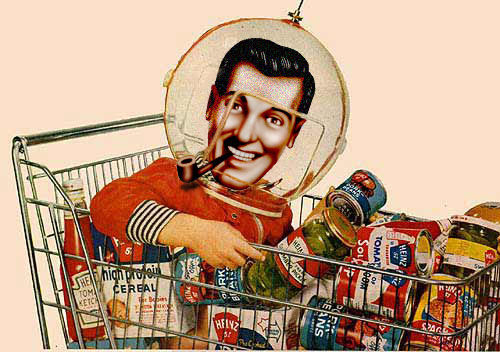There is no shortage of crude, abrasive techniques designed to shock the assuming viewer into a state of submissive attention.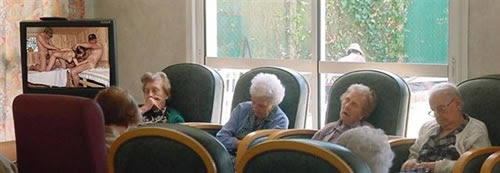: > :;(:(:0;)::(:(:>:)(:)(<:((),0(<)9)((:):())(:)(:)(:):():()):(:():():(:&(&\$^%%^^@(%@(^%:\$!*&@%>>>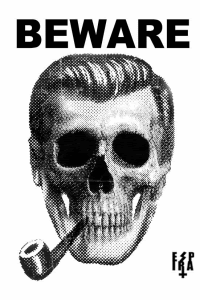So nowadaze, the potty trained Consumer no longer notices the drastic nuances of play at play in the commercial mediascape we are so stealthily bombarded with.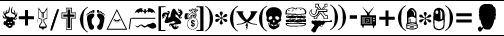They forget that they are responsible for their own Responses. ( Inconclusively, how do You sUbvert commercial subvertizing without just reselling the "goods"?
- (((((((((())))))))))) +